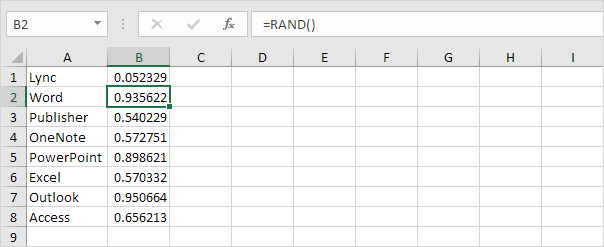# Randomize List

This article teaches you how to randomize (shuffle) a list in Excel. For example, we want to randomize the list in column A below.

1. Select cell B1 and insert the RAND() function.

2. Click on the lower right corner of cell B1 and drag it down to cell B8.3. Click any number in the list in column B.

4. To sort in descending order, on the Data tab, in the Sort & Filter group, click ZA.Result. A random list in column A (sorted on the random numbers above).Note: random numbers change every time a cell on the sheet is calculated. If you don’t want this, simply copy the random numbers and paste them as values.

Next Chapter: Filter